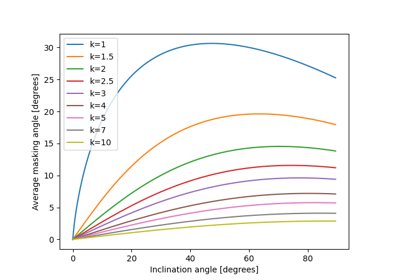pvlib.irradiance.isotropic(surface_tilt, dhi)[source]

Determine diffuse irradiance from the sky on a tilted surface using the isotropic sky model.

$I_{d} = DHI \frac{1 + \cos\beta}{2}$

Hottel and Woertz’s model treats the sky as a uniform source of diffuse irradiance. Thus the diffuse irradiance from the sky (ground reflected irradiance is not included in this algorithm) on a tilted surface can be found from the diffuse horizontal irradiance and the tilt angle of the surface.

Parameters
• surface_tilt (numeric) – Surface tilt angle in decimal degrees. Tilt must be >=0 and <=180. The tilt angle is defined as degrees from horizontal (e.g. surface facing up = 0, surface facing horizon = 90)

• dhi (numeric) – Diffuse horizontal irradiance in W/m^2. DHI must be >=0.

Returns

diffuse (numeric) – The sky diffuse component of the solar radiation.

References

1

Loutzenhiser P.G. et. al. “Empirical validation of models to compute solar irradiance on inclined surfaces for building energy simulation” 2007, Solar Energy vol. 81. pp. 254-267

2

Hottel, H.C., Woertz, B.B., 1942. Evaluation of flat-plate solar heat collector. Trans. ASME 64, 91.

## Examples using pvlib.irradiance.isotropic¶